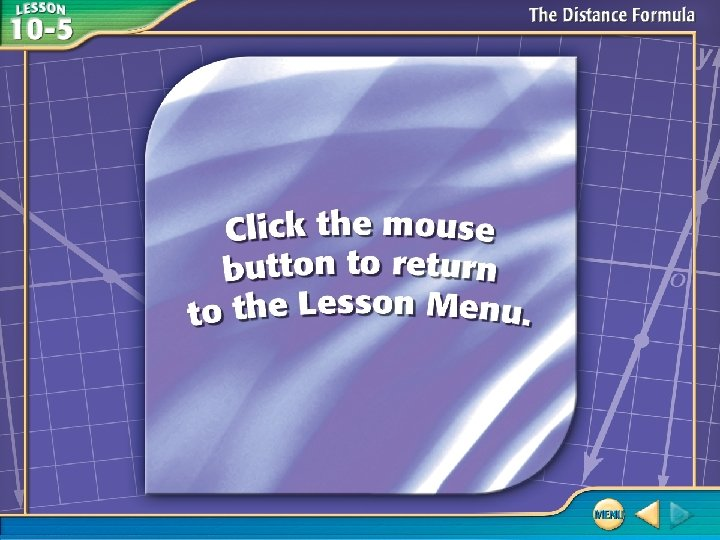Скачать презентацию Five-Minute Check over Lesson 10 4 Then Now New

• Количество слайдов: 25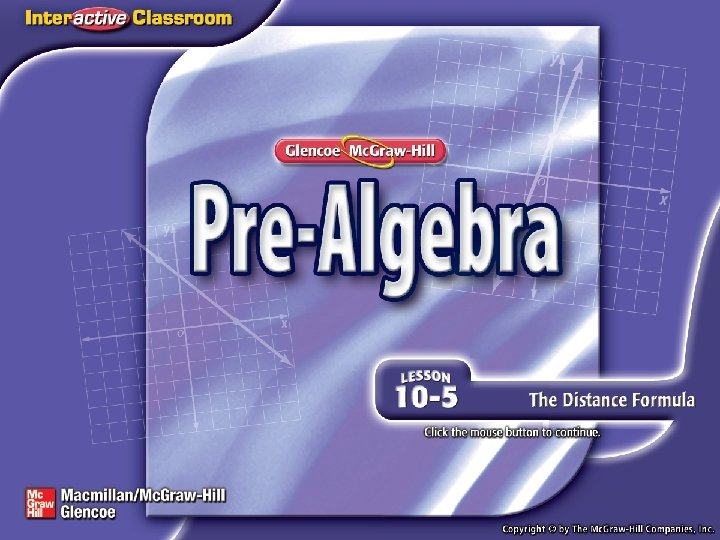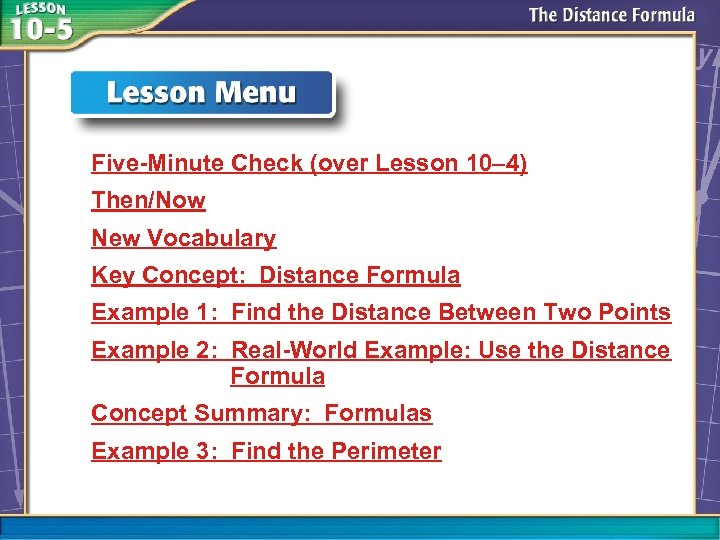Five-Minute Check (over Lesson 10– 4) Then/Now New Vocabulary Key Concept: Distance Formula Example 1: Find the Distance Between Two Points Example 2: Real-World Example: Use the Distance Formula Concept Summary: Formulas Example 3: Find the Perimeter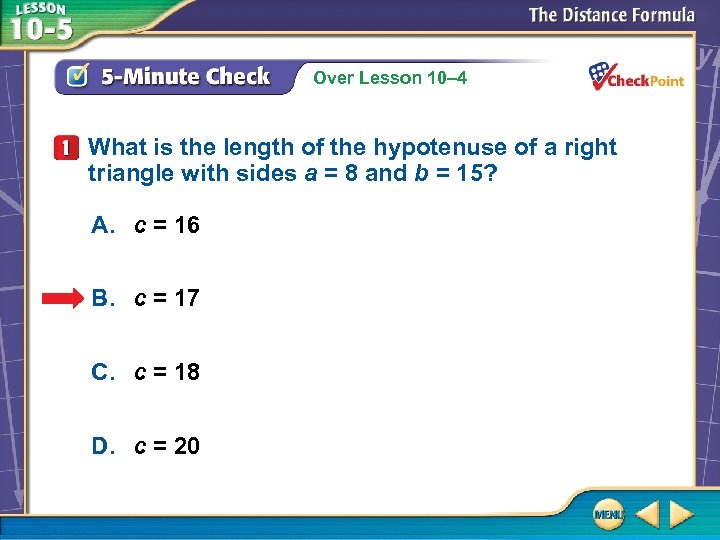Over Lesson 10– 4 What is the length of the hypotenuse of a right triangle with sides a = 8 and b = 15? A. c = 16 B. c = 17 C. c = 18 D. c = 20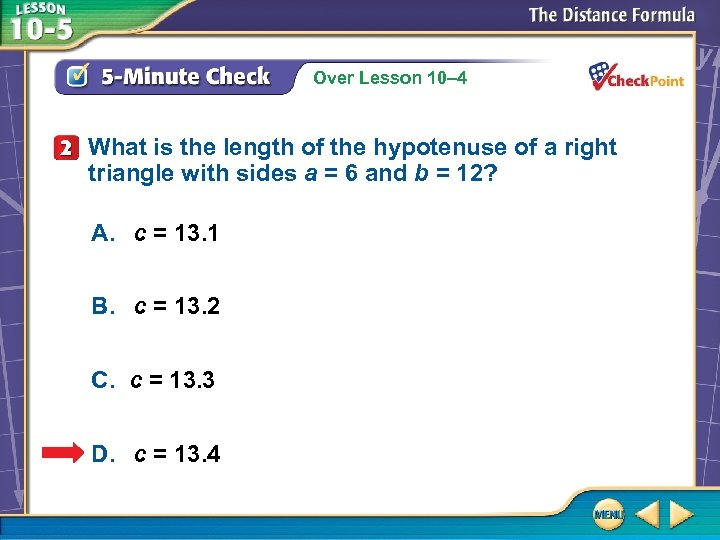Over Lesson 10– 4 What is the length of the hypotenuse of a right triangle with sides a = 6 and b = 12? A. c = 13. 1 B. c = 13. 2 C. c = 13. 3 D. c = 13. 4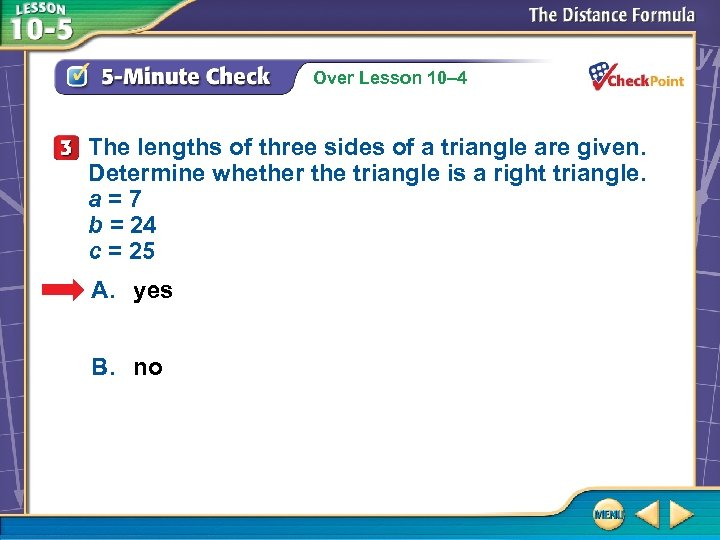Over Lesson 10– 4 The lengths of three sides of a triangle are given. Determine whether the triangle is a right triangle. a=7 b = 24 c = 25 A. yes B. no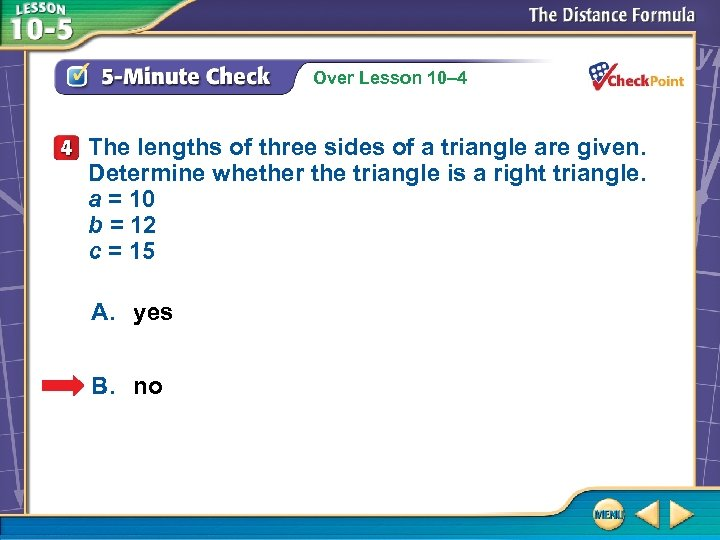Over Lesson 10– 4 The lengths of three sides of a triangle are given. Determine whether the triangle is a right triangle. a = 10 b = 12 c = 15 A. yes B. no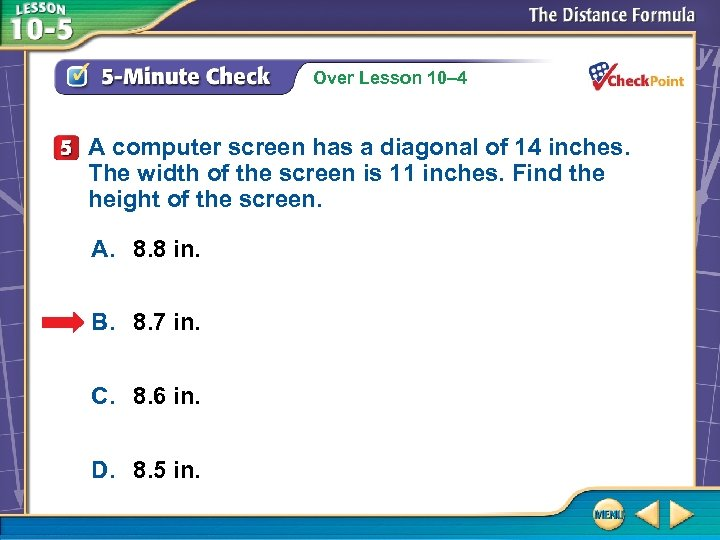Over Lesson 10– 4 A computer screen has a diagonal of 14 inches. The width of the screen is 11 inches. Find the height of the screen. A. 8. 8 in. B. 8. 7 in. C. 8. 6 in. D. 8. 5 in.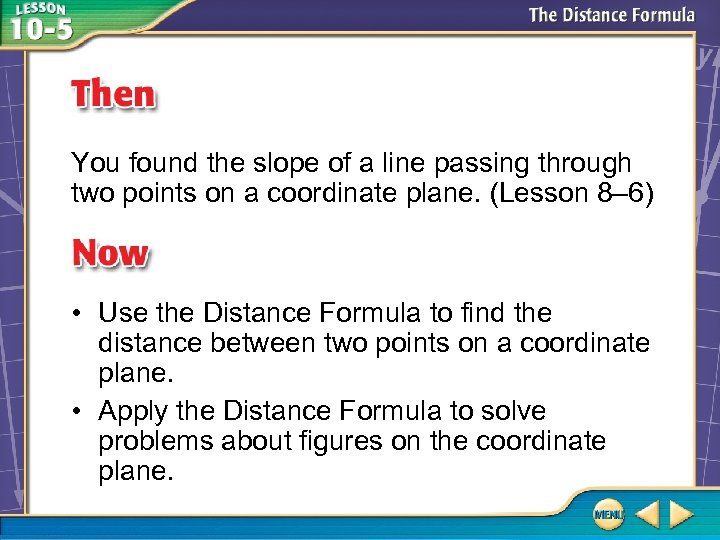You found the slope of a line passing through two points on a coordinate plane. (Lesson 8– 6) • Use the Distance Formula to find the distance between two points on a coordinate plane. • Apply the Distance Formula to solve problems about figures on the coordinate plane.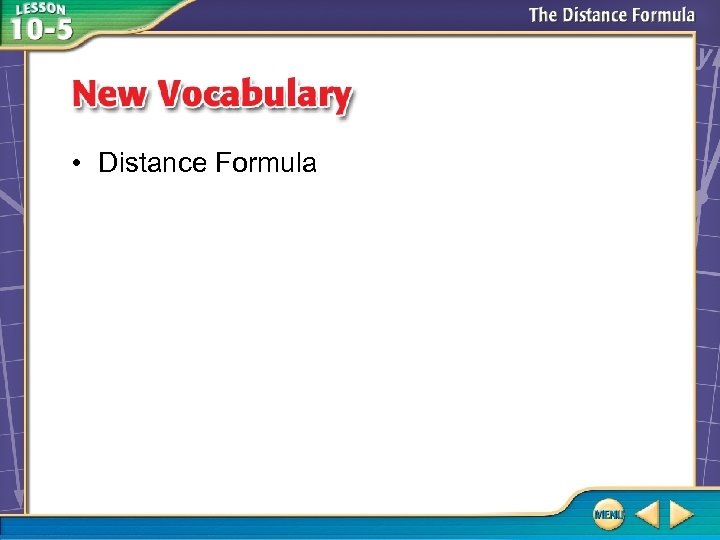• Distance Formula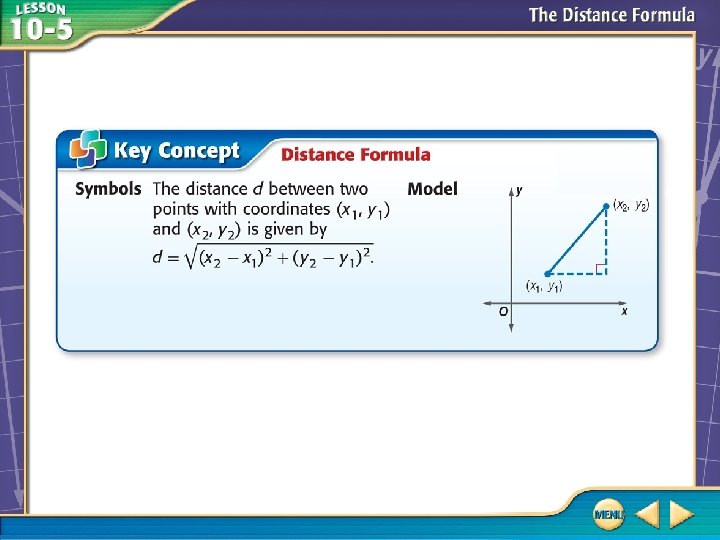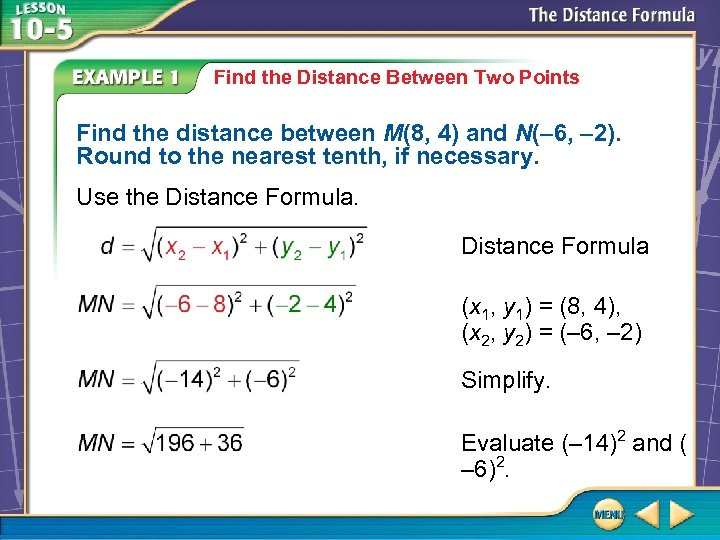Find the Distance Between Two Points Find the distance between M(8, 4) and N(– 6, – 2). Round to the nearest tenth, if necessary. Use the Distance Formula (x 1, y 1) = (8, 4), (x 2, y 2) = (– 6, – 2) Simplify. Evaluate (– 14)2 and ( – 6)2.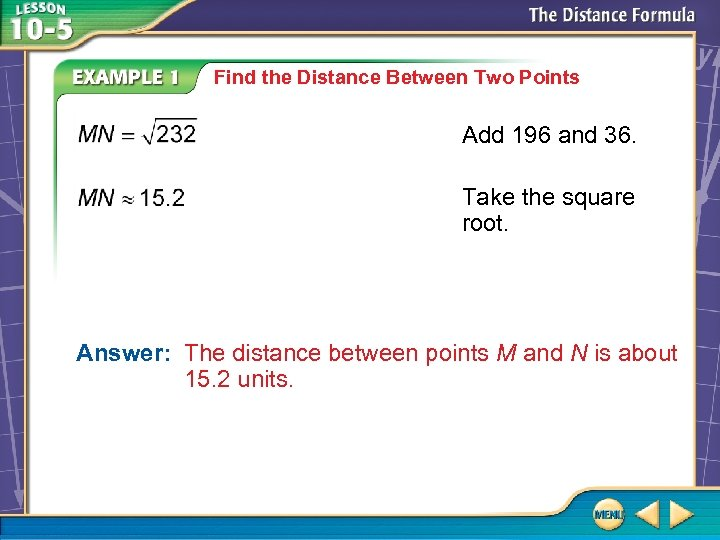Find the Distance Between Two Points Add 196 and 36. Take the square root. Answer: The distance between points M and N is about 15. 2 units.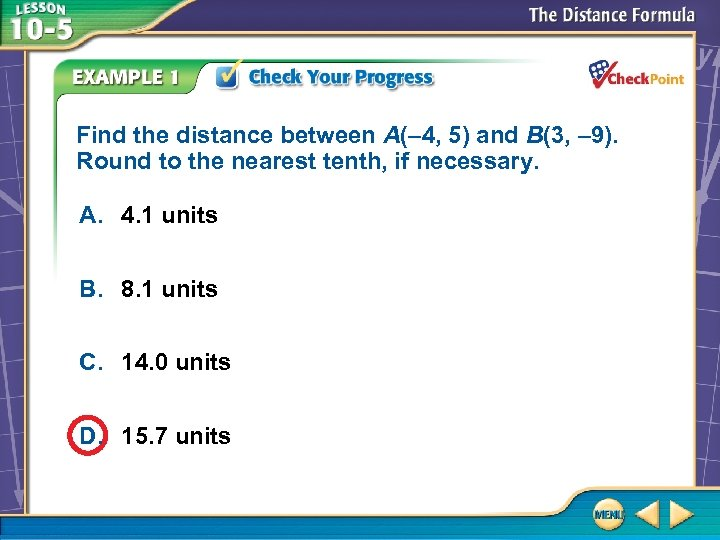Find the distance between A(– 4, 5) and B(3, – 9). Round to the nearest tenth, if necessary. A. 4. 1 units B. 8. 1 units C. 14. 0 units D. 15. 7 units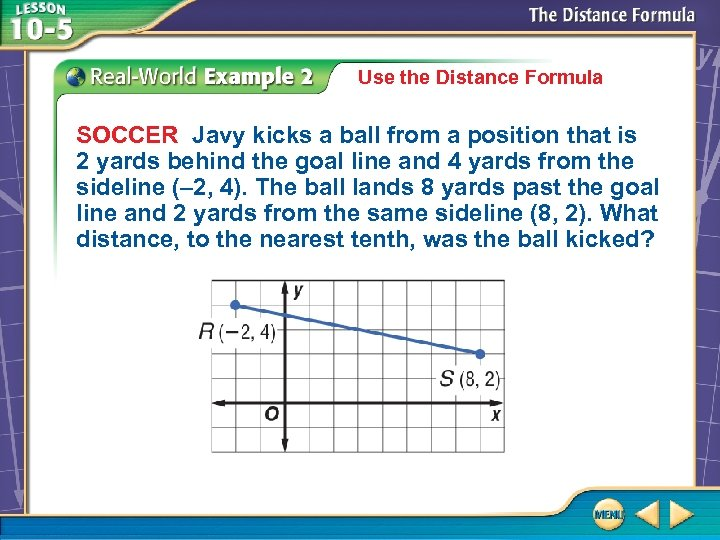Use the Distance Formula SOCCER Javy kicks a ball from a position that is 2 yards behind the goal line and 4 yards from the sideline (– 2, 4). The ball lands 8 yards past the goal line and 2 yards from the same sideline (8, 2). What distance, to the nearest tenth, was the ball kicked?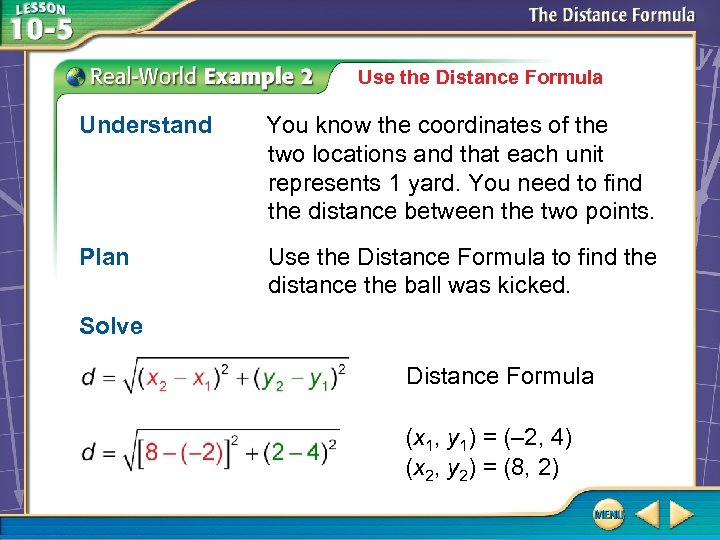Use the Distance Formula Understand You know the coordinates of the two locations and that each unit represents 1 yard. You need to find the distance between the two points. Plan Use the Distance Formula to find the distance the ball was kicked. Solve Distance Formula (x 1, y 1) = (– 2, 4) (x 2, y 2) = (8, 2)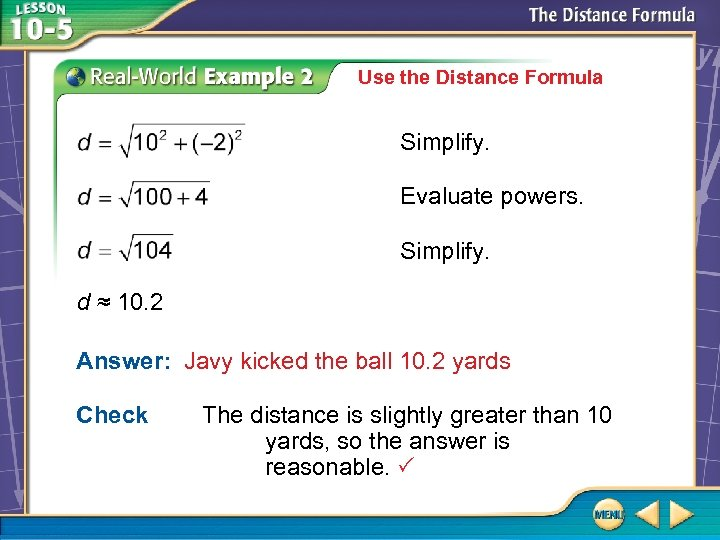Use the Distance Formula Simplify. Evaluate powers. Simplify. d ≈ 10. 2 Answer: Javy kicked the ball 10. 2 yards Check The distance is slightly greater than 10 yards, so the answer is reasonable.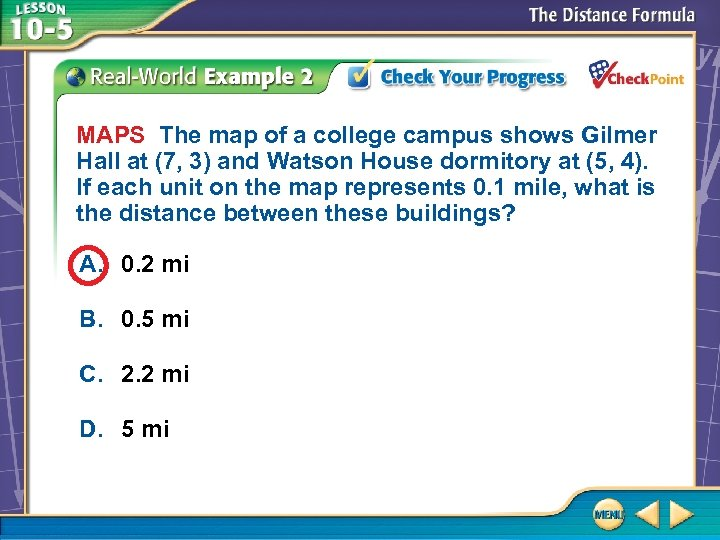MAPS The map of a college campus shows Gilmer Hall at (7, 3) and Watson House dormitory at (5, 4). If each unit on the map represents 0. 1 mile, what is the distance between these buildings? A. 0. 2 mi B. 0. 5 mi C. 2. 2 mi D. 5 mi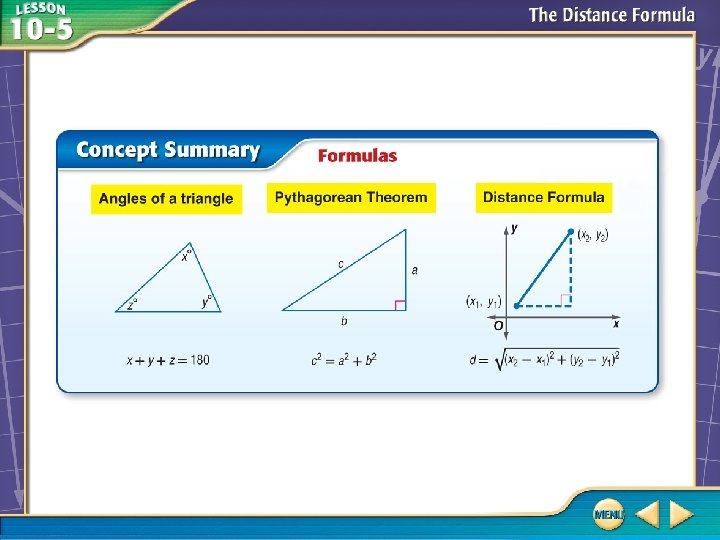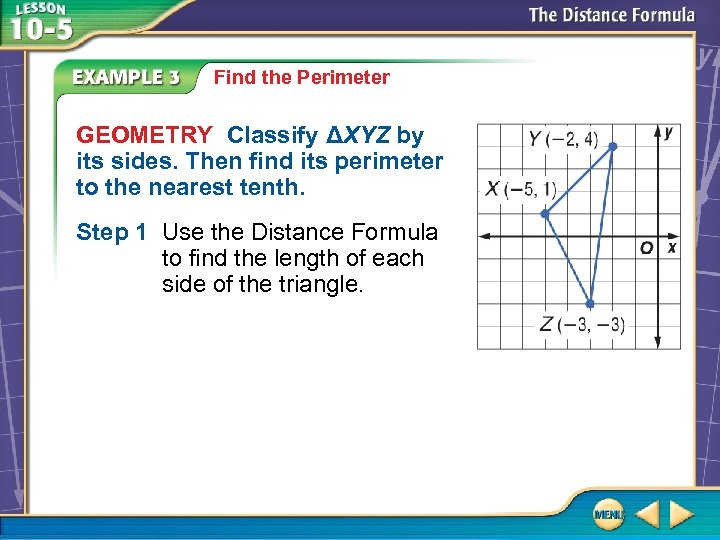Find the Perimeter GEOMETRY Classify ΔXYZ by its sides. Then find its perimeter to the nearest tenth. Step 1 Use the Distance Formula to find the length of each side of the triangle.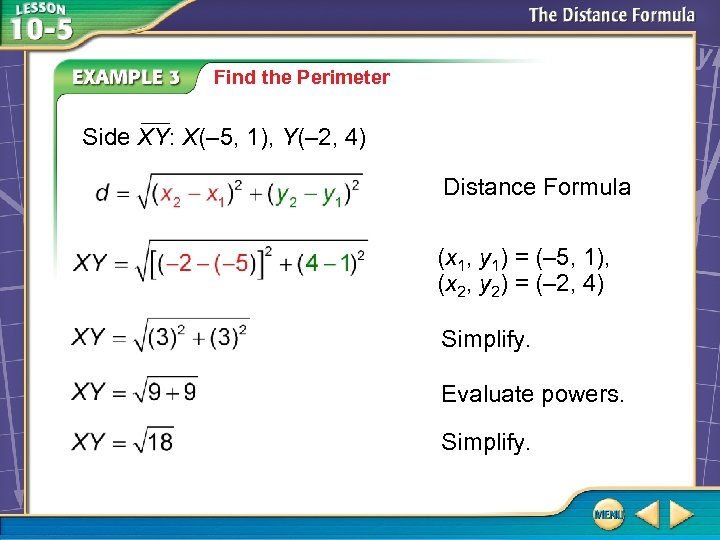Find the Perimeter Side XY: X(– 5, 1), Y(– 2, 4) Distance Formula (x 1, y 1) = (– 5, 1), (x 2, y 2) = (– 2, 4) Simplify. Evaluate powers. Simplify.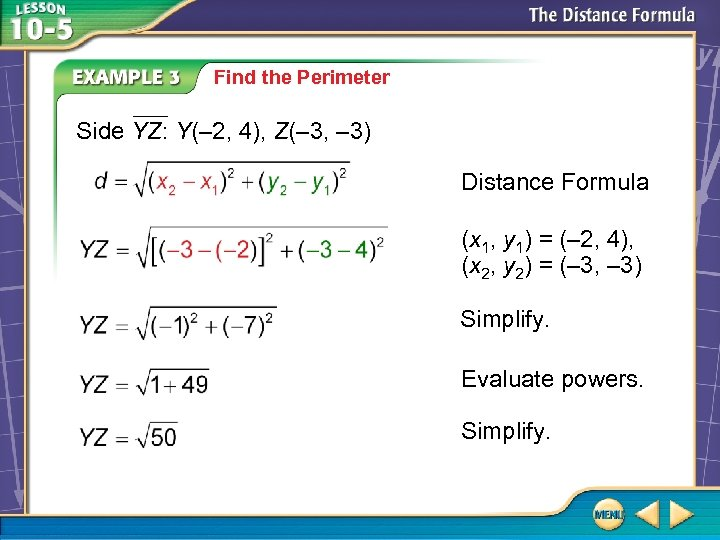Find the Perimeter Side YZ: Y(– 2, 4), Z(– 3, – 3) Distance Formula (x 1, y 1) = (– 2, 4), (x 2, y 2) = (– 3, – 3) Simplify. Evaluate powers. Simplify.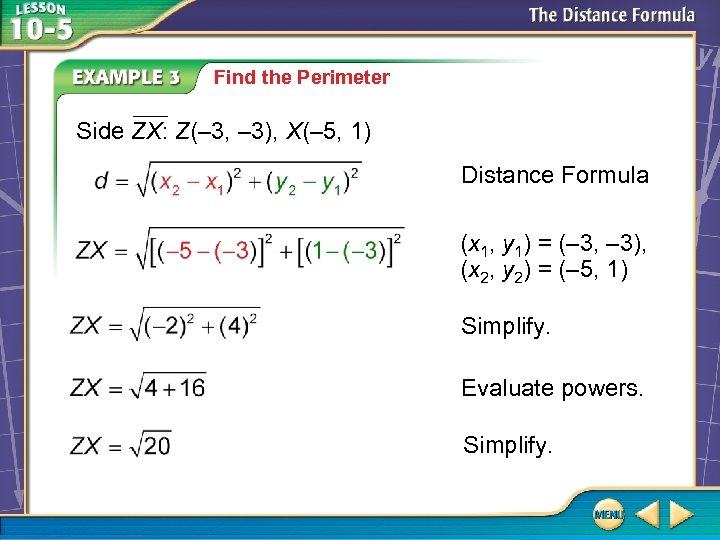Find the Perimeter Side ZX: Z(– 3, – 3), X(– 5, 1) Distance Formula (x 1, y 1) = (– 3, – 3), (x 2, y 2) = (– 5, 1) Simplify. Evaluate powers. Simplify.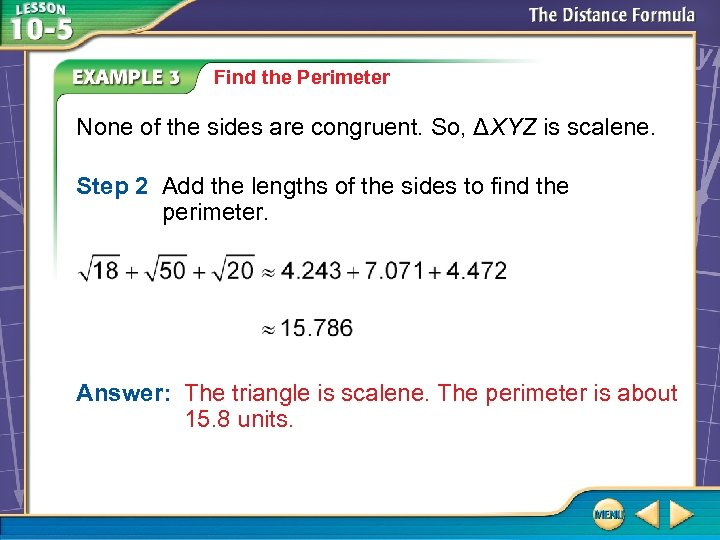Find the Perimeter None of the sides are congruent. So, ΔXYZ is scalene. Step 2 Add the lengths of the sides to find the perimeter. Answer: The triangle is scalene. The perimeter is about 15. 8 units.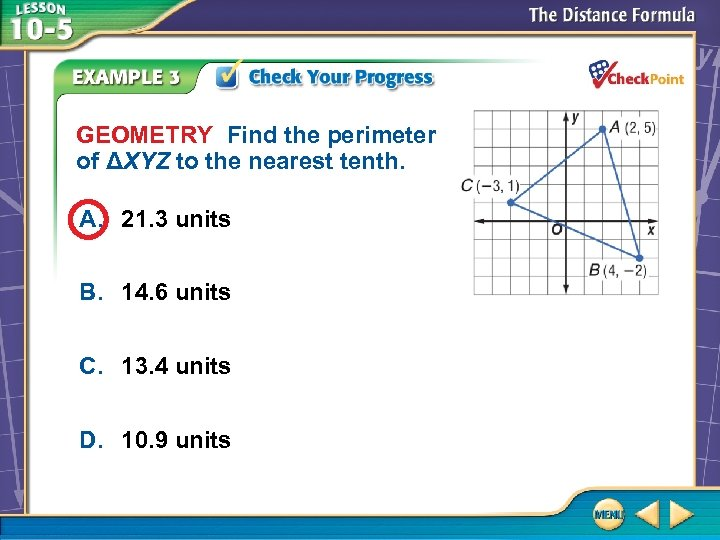GEOMETRY Find the perimeter of ΔXYZ to the nearest tenth. A. 21. 3 units B. 14. 6 units C. 13. 4 units D. 10. 9 units